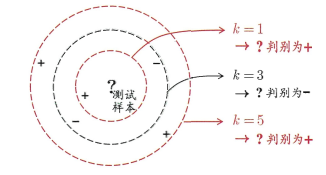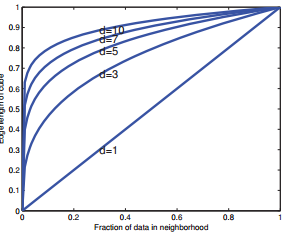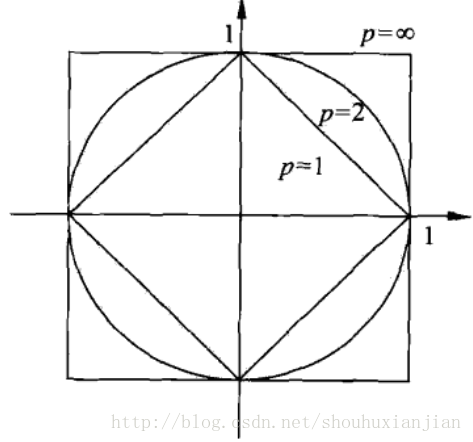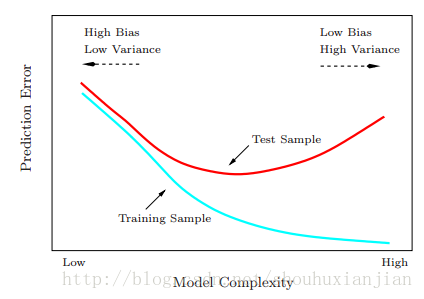# KNN-笔记(1)

### 1 - 背景

KNN：k近邻，表示基于k个最近的邻居的一种机器学习方法。该方法原理简单，构造方便。且是一个非参数化模型。
KNN是一个“懒学习”方法，也就是其本身没有训练过程。只有在对测试集进行结果预测的时候才会产生计算。KNN在训练阶段，只是简单的将训练集放入内存而已。该模型可以看成是对当前的特征空间进行一个划分。当对测试集进行结果预测时，先找到与该测试样本最接近的K个训练集样本，然后基于当前是分类任务还是回归任务来做对应的处理。

KNN模型中有三个需要注意的地方：
1 - 距离度量的方法；
2 - K值的选择；
3 - 最后的判别决策规则。

1）分类任务，那么找这K个训练集样本中出现次数最多的那个标签作为该测试样本标签，如下图：2）回归任务，基于这K个训练集样本求均值，将其作为该测试集样本的结果。

$e_D(f) = f^{1/D}$不过幸运的是， 有一个效应可以在一定程度上抵消维度灾难， 那就是所谓的“ 非均匀性的祝福”（blessing of nonuniformity） 。



### 2 距离度量

KNN中最常用的方法就是欧式距离计算法，当然也有$L_p$距离和马氏距离等等。

$L_p^{(x_i,x_j)}=(\sum_{l=1}^n|x_i^{l}-x_j^{l}|^p)^{\frac{1}{p}}$

$p=2$时，称为欧式距离；
$p=1$时，称为曼哈顿距离；
$p=\infty$时，是各个坐标距离的最大值，即：

$L_\infty(x_i,x_j)={max}_l|x_i^{(l)}-x_j^{(l)}|$### 3 K值选取

K值的选择会对KNN模型的结果产生重大影响。这就是一个模型选择问题。

$\begin{eqnarray} EPE_k^{(x_0)} &=& E[(Y-\hat f_k(x_0))^2|X=x_0]\\ &=& \sigma^2+[Bias^2(\hat f_k(x_0))+Var(\hat f_k(x_0))]\\ &=& \sigma^2+[f(x_0)-\frac{1}{k}\sum_{l=1}^kf(x(l))]^2+\frac{\sigma^2}{k} \end{eqnarray}$### 4 搜索优化

#### 4.1 - KD树

##### 4.2 - Ball树

(待续)

[] Machine Learning A Probabilistic Perspective
[] 李航，统计学习方法
[] The Elements of Statistical Learning Data Mining, Inference, and Prediction (Second Edition)
[] Pedro Domingos,A Few Useful Things to Know About Machine Learning
[] 以叶子为数据的http://www.cnblogs.com/lysuns/articles/4710712.html
[] http://blog.csdn.net/likika2012/article/details/39619687

posted @ 2018-10-11 16:29  仙守  阅读(272)  评论(0编辑  收藏  举报